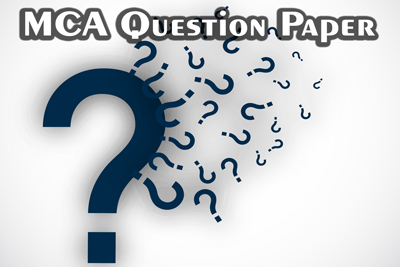WBUT : MCA 5th Semister - Java - 2011
01-08-2017    1001 timesWBUT or West Bengal university of technology is conducted MCA along with various technical courses. According to their MCA syllabus, students learn JAVA in the 5th semester. We are trying to give some guidance to the WBUT students by previous year solved papers. Here we discuss the Java paper of 2011.

OBJECT ORIENTED PROGRAMMING WITH JAVA
2011

Time Allotted: 3 Hours Full Marks: 70

The figures in the margin indicate full marks. Candidates are required to give their answers in their own words as far as practicable.

GROUP – A
( Multiple Choice Type Questions )

1. Choose the correct alternatives for the following : 10 × 1 = 10

i) What is the result of attempting to compile and run the program ?

public class Test
{
public static void print(Integer arg)
{
System.out.print("Integer");
}
public void print(int arg)
{
System.out.print("int");
}
public static void main(String[] arg)
{
print(new Integer(3));
}//end of main
}//end of test class

a) int b) integer
c) compilation error d) none of these.

ii) What will be the output ?
public class Ques
{
private int i;
public Ques incre()
{
i ++;
return new Ques();
}
public void show()
{
System.out.println("i=" +i);
}
public static void main(String[]args)
{
Ques Q1 = new Ques();
Q1.incre().incre().show();
}
}

a) i = 2 b) i = 3
c) compilation error d) i = 0.

iii) int i = “hello” ; what type of exception java throws ?
a) IllegalArgumentException b) NumberFormatException
c) IntegertoStringCastingException d) CastingException.iv) The method used in Java which waits for the thread to die is
a) wait( ) b) destroy( )
c) die( ) d) join( )

v) Which code declares class B belong to the package1.mypackage
a) package package1 ; package mypackage b) import package1.* ;
c) package package1.mypackage.B ;  d) package package1.mypackage ;

vi) Consider the following statements :

int a=15, b=20;
a=((a<b)?(a+b):(a–b);
What will be the value of aafter executing these statements ?
a) 10 b) 25
c) 15 d) 5
e) error, cannot be executed.

vii) Multiple inheritance is implemented in Java by

a) extending two or more classes
b) extending one class and implementing one or more interfaces
c) implementing two or more interfaces
d) all of these.

viii) Difference between String and StringBuffer class is
a) String object can be extendable but StringBuffer object cannot be extendable
b) String object can be extendable but StringBuffer object can be expandable
c) both are same
d) none of these.

ix) What is immediate superclass of applet class ?
a) Panel b) Object
c) Interface d) None of these.

x) super() is used to
a) access superclass constructor within subclass constructor
b) access superclass method within subclass constructor
c) both (a) and (b)
d) none of these.

GROUP – B
( Short Answer Type Questions )

Answer any three of the following. 3X5 = 15
2. Create an array that holds the integer, string, double information.

Ans.

3. What is the checked and unchecked exception ? Explain with proper examples.

Ans.

4. What is JVM ? Java is platform-independent language. -Explain. 2 + 3

Ans.

5. What is polymorphism ? What are the types of polymorphism ?2 + 3

Ans.

6. What is threading ? Explain how you can synchronize multiple threads in Java ?

Ans.

GROUP – C
( Long Answer Type Questions )

Answer any threeof the following. 3X15 = 45

7. a) Write a program that takes a file name and a search string from the user. If the search string occurs in the file, then it counts the number of occurrences of the string. ( Assume that search pattern can exist more than two times in a line ).

Ans.

b) How to call a constructor from another one ?

Ans.

c) If we define the constructor in the private section then how can we create an object of that class ?

Ans.

d) “In Java the variables are initialized before any method called even constructor.” Explain with an example. 8 + 2 + 2 + 3

Ans.

8. a) Write a program that stores information in the array and then sort the array.

Ans.

b) What is the difference between the character stream and byte stream ?

Ans.

c) How does Java garbage collector work ?

Ans.

d) What is the object serialization in Java ? Illustrate with an example. 4 + 3 + 3 + 5

Ans.

9. a) What is the difference between method overloading and method overriding ? Illustrate with an example.

Ans.

b) Write a Java class ComLineTest to print the number of arguments passed on the command line as well as the 1st letter of the arguments. For example, if the command line arguments are “Simple Object Robust” the output will be No. of arguments-3 SOR Write a main method to test the class.

Ans.c) How do we define try and catch block ? 4 + 8 + 3

Ans.

10. a) What is exception ? How can you handle the exception in Java ? Explain.

Ans.

b) What is user-defined exception ? Explain with a suitable program.

Ans.

c) What is the use of “finally” keyword ? ( 1 + 5 ) + ( 2 + 5 ) + 2

Ans.

11. Write short notes on any threeof the following : 3 x 5
a) Abstraction

b) Exception handler

c) Encapsulation

d) Container class

e) Inheritance.